# Design and Analyze Parabolic-Reflector-Backed Wideband Eggcrate Array

This example shows how to create an eggcrate array of `vivaldiOffsetCavity` antenna elements backed by a parabolic reflector. The Vivaldi antenna is widely used in radar and electronic countermeasures because of its simple structure and wide operating bandwidth. The eggcrate array consists of Vivaldi antenna elements oriented in a grid. The eggcrate array in Antenna Toolbox™ is a fully metallic array, which is capable of handling a high average power. Vivaldi phased arrays are used for a wide range of applications at various frequencies and bandwidths. The eggcrate array of vivaldi antenna elements provides a wide bandwidth. You can back the array with any Antenna Toolbox backing structure. In this example, you analyze the Vivaldi element, eggcrate array, and eggcrate array backed with a parabolic reflector over 5G frequency bands.

### Create Vivaldi Offset Cavity Antenna

The all-metal vivaldi antenna is lightweight, has a high structural strength, and is suitable for airborne applications. The antenna consists of a tapered slot, a feed slot, and a rectangular cavity.

Create a `vivaldiOffsetCavity` antenna element by using the `design` function. Choose a 5G frequency of 26 GHz to perform analysis on the antenna element and the eggcrate array.

```vc = vivaldiOffsetCavity; freq = 26e9; lambda = physconst("lightspeed")/26e9; ant = design(vc,freq);```

Plot the S-parameters of the antenna element over the 25 to 34 GHz frequency range. The magnitude of the reflection coefficient is less than -10 dB over the 27 to 33.9 GHz frequency range, so the antenna has a bandwidth of 6.9 GHz.

```freqRange = linspace(25e9,34e9,100); s = sparameters(ant,freqRange); figure rfplot(s)```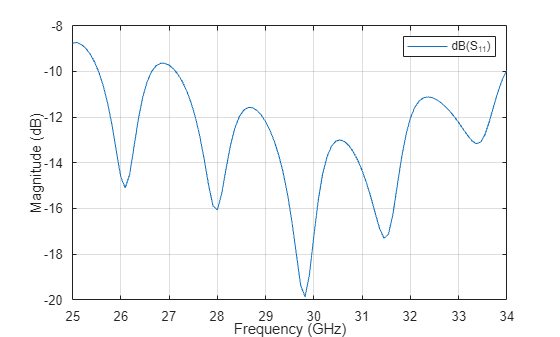Plot the radiation pattern of the antenna at 26 GHz. The maximum gain of the antenna element is 8.55 dB.

```freq = 26e9; figure; pattern(ant,freq);```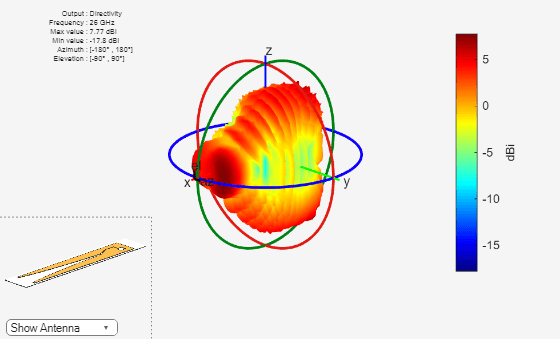### Create and Analyze Eggcrate Array

Create an eggcrate array with offset Vivaldi cavity elements. You can also change the element of the eggcrate array to a Vivaldi notch antenna on a ground plane. The default eggcrate array size is 2-by-2. The total number of elements in an eggcrate of size $N$-by-$N$ is $2{N}^{2}$, so a 2-by-2 array contains eight elements. You can also create a linear array of Vivaldi antennas by defining the size of the eggcrate array as 1-by-$N$ or $N$-by-1.

```earray = eggCrate; earray.Element = ant; figure show(earray)```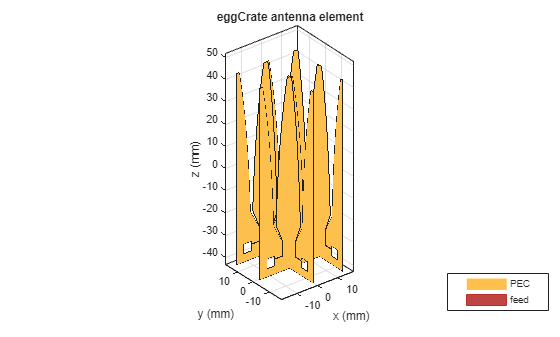Mesh the eggcrate array with a maximum edge length equal to half the free-space wavelength. You can vary the maximum edge length to make the mesh coarser or denser. The computation time for solving an antenna or array depends on the number of triangles in the mesh.

```figure mesh(earray,MaxEdgeLength=lambda/2);```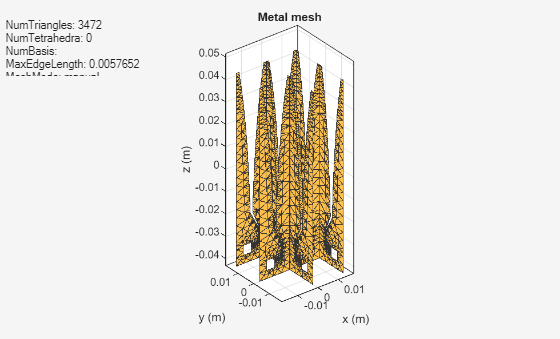Compute and plot the S-parameters of the eggcrate array over the 24 to 34 GHz frequency range. The magnitude of the reflection coefficient is less than -10 dB between 25.6 and 31.6 GHz.

```freqRange = linspace(24e9,34e9,100); s = sparameters(earray,freqRange); figure for i = 1:earray.NumElements hold on rfplot(s,i,i) end lg = legend(Location="bestoutside");```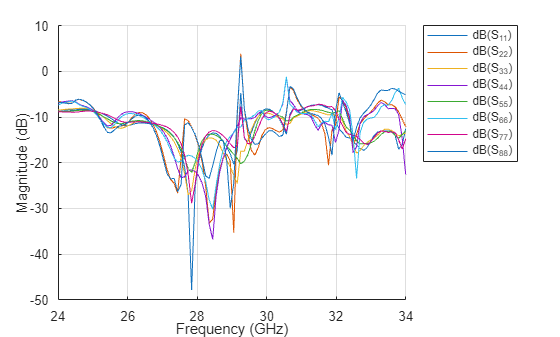Plot the radiation pattern of the eggcrate array at 26 GHz. The maximum gain of the eggcrate array at 26 GHz is 11.4 dB.

```figure pattern(earray,freq)```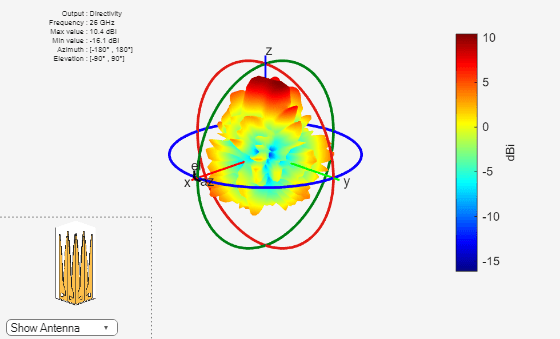### Create and Analyze Eggcrate Array Backed with Parabolic Reflector

Design the parabolic reflector at 26 GHz and position the eggcrate array at a focal length of 15.5λ, where λ is the free-space wavelength. Choose the method of moments (MOM) and physical optics (PO) hybrid solver (MoM-PO). Orient the eggcrate array towards the backing reflector.

```earray.Tilt = 180; rp = design(reflectorParabolic,26e9); rp.Exciter = earray; rp.SolverType = "MoM-PO"; rp.FocalLength = 15.5*lambda; figure show(rp)```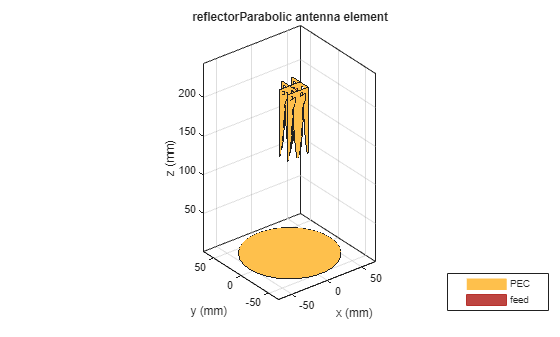Compute and plot the reflection coefficients of each element of the array over the 25 to 33 GHz frequency range. The magnitude of the reflection coefficients is less than -10 dB between 25.8 and 31.7 GHz.

```freqRange = linspace(25e9,33e9,150); srp = sparameters(rp,freqRange); figure for i = 1:earray.NumElements hold on rfplot(srp,i,i) end lg = legend(Location="bestoutside");```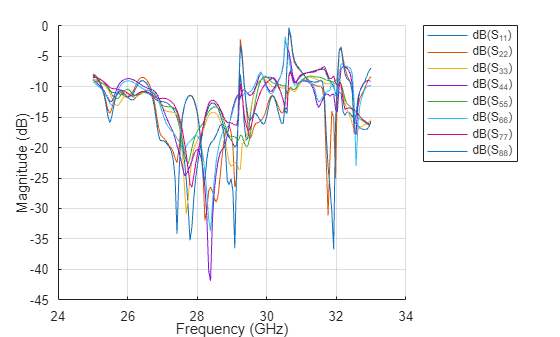Plot the radiation pattern of parabolic reflector backed eggcrate array at 26 GHz.

```figure pattern(rp,freq)```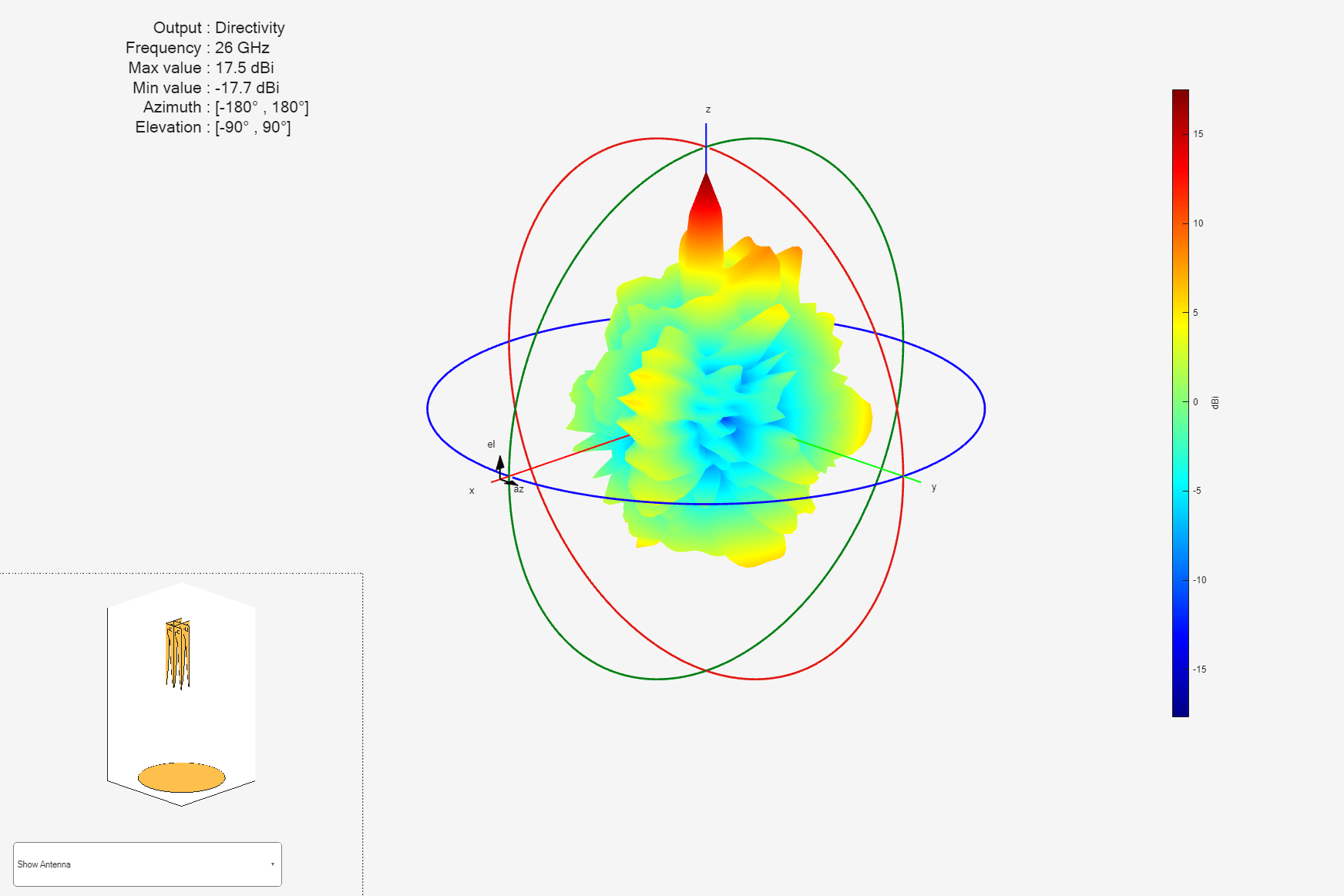### Conclusion

The reflection coefficient of the eggcrate array and the parabolic-reflector-backed eggcrate array are less than -10 dB in the 25.8 to 31 GHz frequency range. The gain of the eggcrate array is 11.5 dB and increases to 17.1 dB when you back it with a parabolic reflector. You can further explore the radiation pattern behavior by varying the design parameters like the focal length of the reflector and the size of the eggcrate array.

### References

 Ma, Xin, Shunlian Chai, Ke Xiao, and Liang Ding. “Design of All-Metal Vivaldi Phased Array Antenna.” In 2018 IEEE 3rd International Conference on Signal and Image Processing (ICSIP), 547–51. Shenzhen: IEEE, 2018. https://doi.org/10.1109/SIPROCESS.2018.8600487.

 Kindt, Rick W, and William R Pickles. “Ultrawideband All-Metal Flared-Notch Array Radiator.” IEEE Transactions on Antennas and Propagation 58, no. 11 (November 2010): 3568–75. https://doi.org/10.1109/TAP.2010.2071360.